• 最近跟一个出版社签了...Word编辑论文的公式时，有3个核心问题需要解决：需要有办法快速的编辑公式、并公式插入特殊字符；很多时候，公式要居中，而公式的标号需要右对齐；需要正文引用公式的标号。这篇...
最近跟一个出版社签了《数据挖掘算法导论》的出版合同，因为交稿日期并不远，这些天都在认真写字。书中太多公式需要编辑，当然可以装一个MarthType插件，但本着“一事不烦二主”的指导原则，还是想办法用Word解决所有问题。Word编辑论文中的公式时，有3个核心问题需要解决：需要有办法快速的编辑公式、并在公式中插入特殊字符；很多时候，公式要居中，而公式的标号需要右对齐；需要在正文中引用公式的标号。这篇文章就这三个问题，介绍对应比较靠谱的方法。（所用的工作环境是Windows10+Office专业增强版2019）快速插入公式、插入特殊字符你在word中插入公式的时候是怎么做的？是不是总共分三步？首先，把Word打开；然后，点击“插入”；最后，点公式。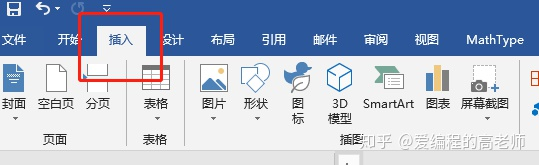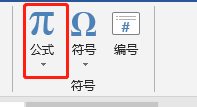三步走完成之后就会在word的光标处会变成下图这样，就可以插入公式了。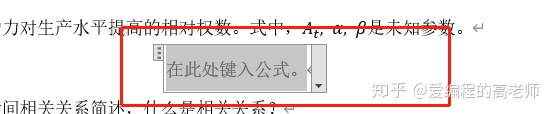但是，有一种更快速的办法可以用快捷键一步就可以插入Word公式，你晓得么？同时按住键盘上的“Alt”键  和“=”键，就可以快速的插入公式。插入公式是公式编辑的第一步，在公式编辑中最恼人的是特殊字符的插入，比如要插入一个怎么搞呢？比较常规的做法是，在公式编辑环境下点击“设计”选项卡，然后在符号区域点下拉，找到并点击完成插入。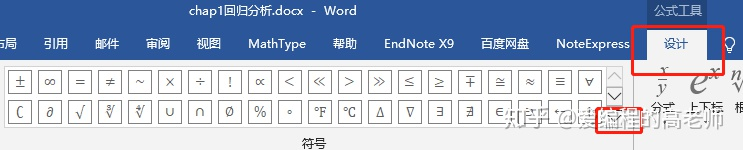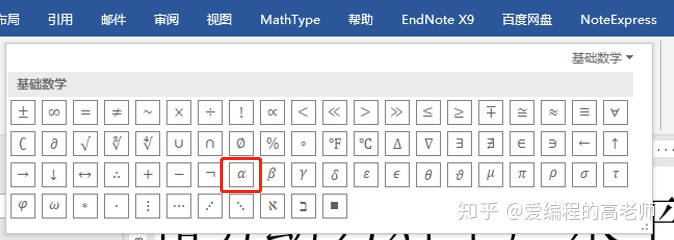除此之外，还有一种更好的办法，在公式编辑的时候输入"alpha”，然后按一下键盘上的空格键，就可以完成的编辑，如下面的图。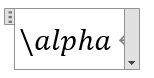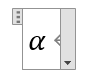除了特殊字符的编辑，像下角标啊、上角标啊也可以用类似的方法来进行编辑。比如“x_1”然后按一下空格键，就可以实现下标输入；“y^(x+y)"就可以实现上标输入。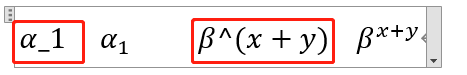戴帽子等等，都可以使用这种方式来进行编辑。需要注意的是，此时公式的转换的地方的格式是/ Unicode的。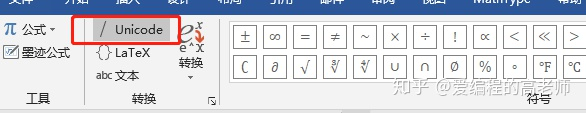此时，还有一个问题，当我们使用上面的办法插入特殊字符的时候老是记不住怎么办呢？不用着急，当你采用传统方法插入特殊字符时，如果把鼠标移动到字符处稍微停顿一下就会出现这个字符的unicode输入码的提示。如下图：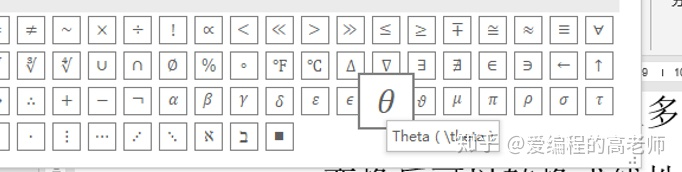当然，我这篇文章只是告诉你Word公式编辑中有类似Latex公式编辑的Unicode公式编辑方法，更具体的教程你可以在网上搜索”Word Unicode Math编码教程“学习一下。如果你需要经常使用word编辑公式的话，掌握了unicode的公式编辑方法可以极大提高工作效率。公式样式设计第二个要解决的问题，公式的格式。这也是挺恼人的，很多时候我们要求公式居中，公式的编号居右，这怎么做呢？一种比较容易想到的办法，就是插入一个一行两列的表格，然后再把表格左边的列设为居中、右边的列设为居右，最后把表格的框线隐藏。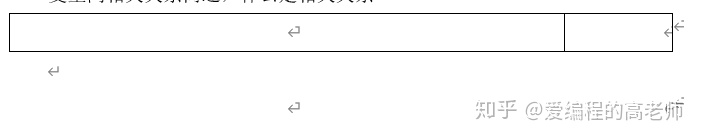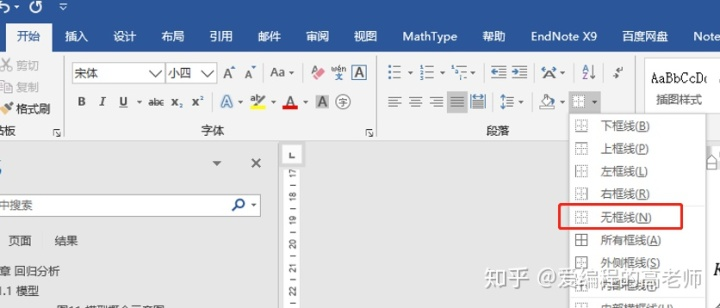这种办法当然也不是不行，只是这样做出来的吧，看上去会怪怪的。而且吧，每次编辑公式都需要插入表格啊什么的，太繁琐！还有一种更高级的办法，刚开始看上去麻烦一点，但等后面就是选中公式，点击一下就对应样式就齐活了。第一步：可以在”开始“选项卡，找到”样式“区，然后点击下拉，再点击样式新建一个名为”公式的样式“。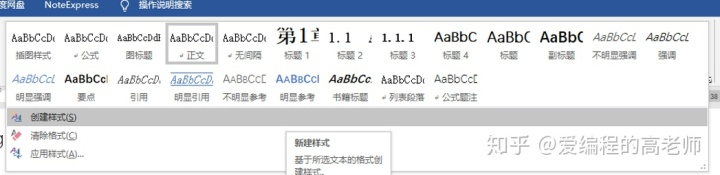第二步：在格式名称中输入”公式“，然后点击”修改“按钮。如下图：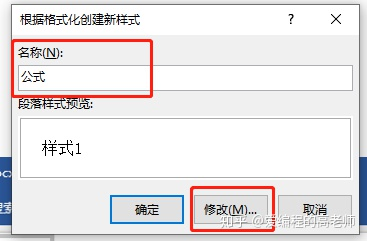第三步：在跳出的格式创建对话框中，点击下面的格式按钮然后选中”制表位“菜单，如下图：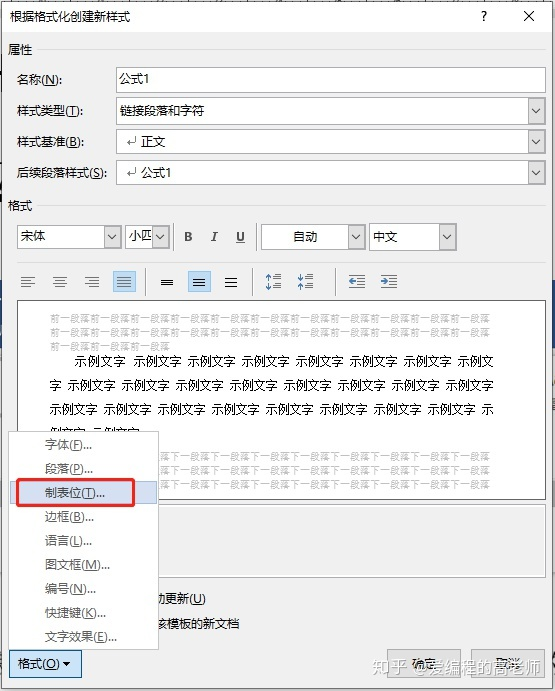第四步：在弹出的制表位对话框中，在制表位位置输入框中输入制表位然后点击下面的”设置“按钮完成制表位添加，如果是A4页大小的话，就如下图所示的字符位置。分别设置为居中对齐、右对齐。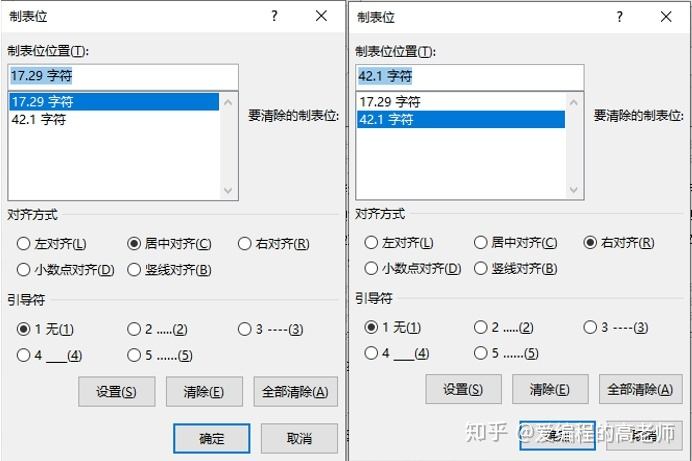第五步：在输入公式的时候，在公式的前面按一下”Tab“键，公式后面再按一下”Tab“键，然后输入公式标号。最后，选中公式这一行，将样式设置为刚刚添加的公式样式即可。如下图。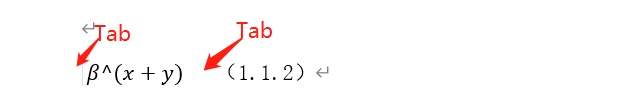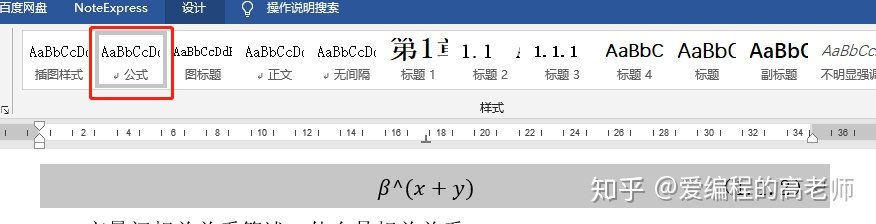公式编号完成公式编辑、样式设置之后呢？还有个麻烦事，就是我们希望对公式自动编号，然后在文中引用公式的时候，还能根据编号的变化自动变化。在Word中可以通过”引用“选项卡中的”插入题注“、”交叉引用“来实现这一目标。但是，在交叉引用的时候有一个小技巧。使用”插入题注“来实现公式的自动编号，依次点击”引用“、”插入题注“ 调出”题注“对话框。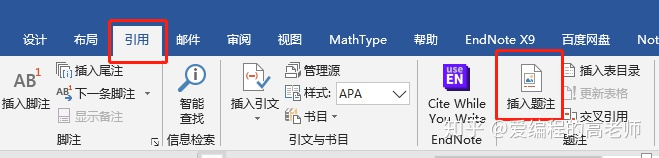然后，点击”新建标签“按钮在”新建标签“对话框中的标签编辑框中输入公式，勾选”从题注中排除标签“复选框。如下图：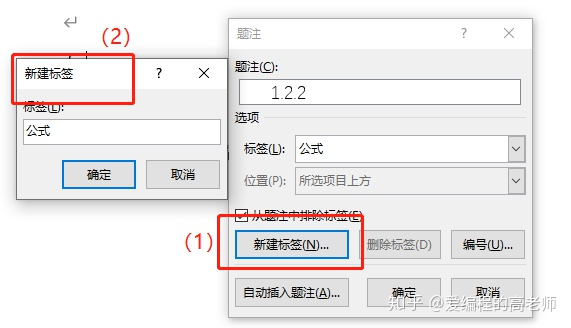完成标签创建后，点击”编号“按钮调出”题注编号“对话框，根据需要选择章节起始样式、使用分隔符（可以是点、中划线等），然后点击”包含章节“复选框，如下图。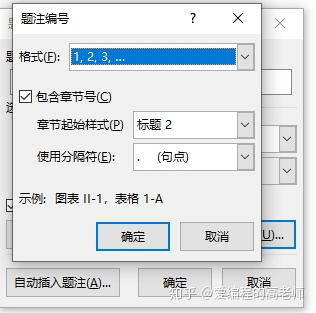完成之后，点击题注对话框中的确定按钮，在光标处就会自动插入包含了二级标题序号的公式编号。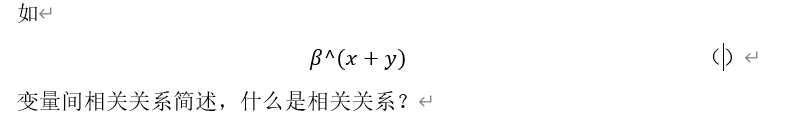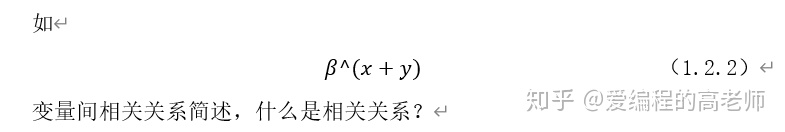完成公式编号后，还需要在正文中必要的位置插入公式编号的引用，如下图：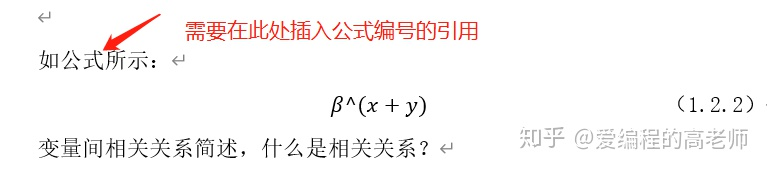可以点击”引用“选项卡中的”交叉引用“，如下图：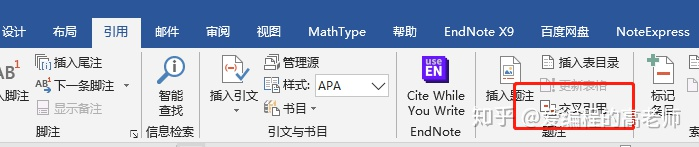弹出”交叉引用“对话框，在引用类型下拉菜单中选择公式，然后在引用哪一个题注的列表中下拉找到刚刚添加的公式编号，问题来了！！发现不止是编号，整个公式都被显示出来了，而且就这样插入交叉引用的话会出问题，这可如何是好？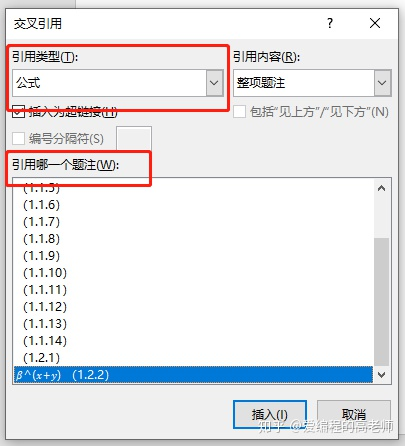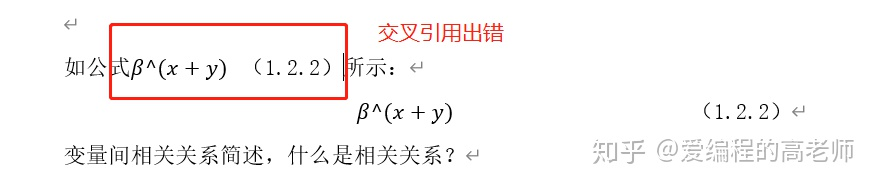这需要对公式那个地方重新来整理一下，在公式的编号前面按一下”Enter“键另起一行，然后光标移回，再同时按键盘上的”Ctrl+Alt+Enter“三个按键，将公式编号移回。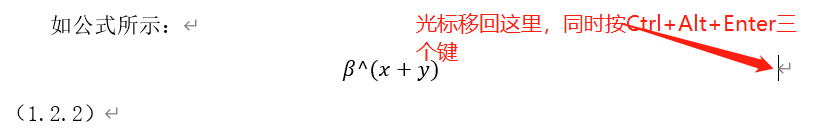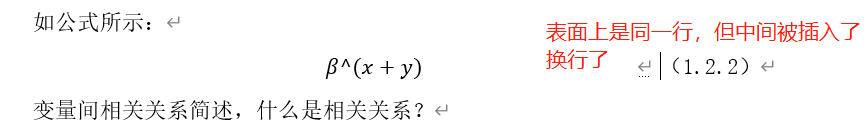这个时候，再打开交叉引用看一下，神奇的事情发生了。再插入交叉引用的时候就不会出错了。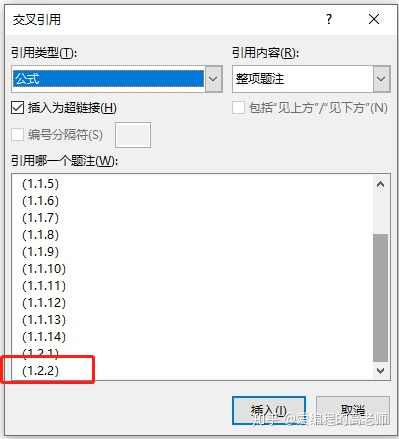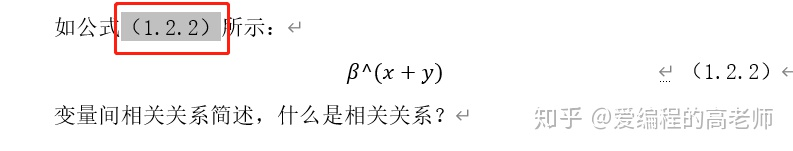总结Word的功能是很强大的，但是有些功能隐藏的很深，想要使用的话必须花心思在网上找找教程、攻略。而且，你正式使用Word编辑一本书、学位论文等等之前，非常有必要学一学这些教程，事半功倍。
展开全文• 在Word文档中某一行使用“Mathtype公式编辑器”输入数学公式后，往往公式所在行...以下步骤教您如何在word中调整公式大小：第一步 在文档中需要插入公式的地方用Mathtype公式编辑器插入需要的公式，可以参考下图在文...
在Word文档中某一行使用“Mathtype公式编辑器”输入数学公式后，往往公式所在行的行距明显变大，就好像公式把这一行和其它行给撑开了一样，非常不美观，使用改变行距的命令也不能解决问题。公式是以图片形式插入word文档的，可以使用缩放图片的形式来调整公式的大小。以下步骤教您如何在word中调整公式大小：第一步 在文档中需要插入公式的地方用Mathtype公式编辑器插入需要的公式，可以参考下图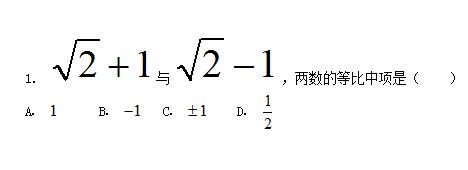在文档中插入所需要的公式第二步 文档中公式与文字相差太大，看起来很不协调，这时我们可以用鼠标点击插入的公式，选中公式，公式周围会出现八个尺寸调控点。示例见下图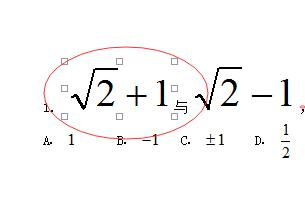选中你所要调整的公式第三步 将鼠标放到尺寸调控点上，当出现双向光标以后，按光标指示的方向拖动鼠标，就可以对公式进行缩放了。示例见下图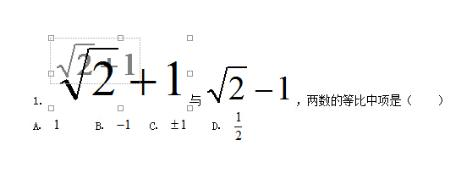局部缩小你选中的公式第四步 如果用鼠标拖动公式四角的某个尺寸控制点，就可以对公式进行整体缩放。示例见下图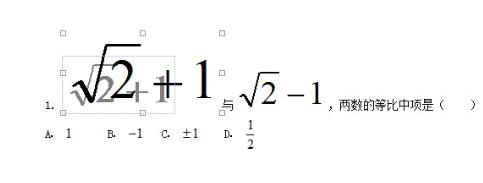整体缩小你选中的公式通过学习以上如何在word中调整公式大小的教程，任何新手用户都可以很快上手，令你的文档看起来更美观。如您还需要其它MathType的快速入门或具体使用技巧技巧可以参考MathType服务中心。
展开全文• 由于近日居家写论文，公式多的一批，用Mathtype或者word自带公式编辑器还要不断的打开关闭插入公式，鼠标点来点去麻烦的很，索性查了一下用latex在word中编辑公式 1、首先避免不了还是需要安装MathTyoe，链接放下边...
近日居家写论文，公式多的一批，用Mathtype或者word自带公式编辑器还要不断的打开关闭插入公式，鼠标点来点去麻烦的很，索性查了一下用LaTeX在word中编辑公式，以下给出过程和自己常用的一些符号公式，以备写公式时使用
一、第一种方法可以使用word自带的公式编辑器：
快捷键“Alt和+”键组合，可在当前位置插入公式，选择LaTeX进行编辑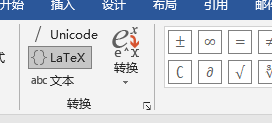如，当输入公式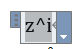点击转换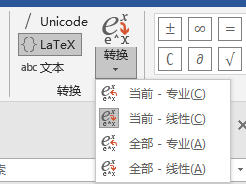可直接转换为公式$z^i$
二、利用MatchType数学公式编辑软件
链接放下边，可以自取，在word中可直接在需要插入公式的位置插入LaTex代码
$数学公式$

然后选中公式，可以通过鼠标点击MathType命令栏中的Toggle Tex按钮，就会自动变成公式。也可以用快捷键Alt+\来完成，按一下变成公式，再按一次回到原来的样子。
MatchType链接：https://pan.baidu.com/s/1QX_ZapwwGDxN-MiUiE-Njg 提取码：74h3

常用特殊符号

LaTeX代码
符号
LaTeX代码
符号

$\alpha$
$\alpha$
$\beta$
$\beta$

$\gamma$
$\gamma$
$\delta$
$\delta$

$\epsilon$
$\epsilon$
$\theta$
$\theta$

$\Theta$
$\Theta$
$\partial$
$\partial$

$\lambda$
$\lambda$
$\mu$
$\mu$

$\xi$
$\xi$
$\pi$
$\pi$

$\rho$
$\rho$
$\sigma$
$\sigma$

$\tau$
$\tau$
$\upsilon$
$\upsilon$

$\phi$
$\phi$
$\chi$
$\chi$

$\psi$
$\psi$
$\omega$
$\omega$

$\carepsilon$
$\varepsilon$
$\varphi$
$\varphi$

$\Omega$
$\Omega$
$\Psi$
$\Psi$

$\Phi$
$\Phi$
$\Sigma$
$\Sigma$

上下标注导数向量等

名称
latex公式
符号

上标
$a^2$ …… $a^{i+j}$
$a^2$ …… $a^{i+j}$

下标
$a_2$ …… $a_{i+j} $a_2$ …… $a_{i+j}$ 上下标$a^i_j$……$a^{i+1}_{j+1}$$a^i_j$ …… $a^{i+1}_{j+1}$ 前置上下标${}^2_1\!X^3_4$${}^2_1\!X^3_4$ 导数$x’$……$\dot{x}$……$\ddot{x}$$x'$ …… $\dot{x}$ …… $\ddot{x}$ 向量$\vec{x}$…$\overleftarrow{AB}$…$\overrightarrow{AB} … $\widehat{AB}$
$\vec{x}$ … $\overleftarrow{AB}$ … $\overrightarrow{AB}$ … $\widehat{AB}$

上划线
$\overline{ABC}$
$\overline{ABC}$

帽
$\hat{a}$…… $\widehat{a}$ …… $\vec{a}$
$\hat{a}$ …… $\widehat{a}$ …… $\vec{a}$

上括号
$\overbrace{1+2+\cdots+100}$
$\overbrace{1+2+\cdots+100}$

……
$\begin{matrix}5050\\\overbrace{1+2+\cdots+100}\end{matrix}$
$\begin{matrix}5050\\\overbrace{1+2+\cdots+100}\end{matrix}$

下括号
$\underbrace{1+2+\cdots+100}$
$\underbrace{1+2+\cdots+100}$

…
$\begin{matrix}\underbrace{1+2+\cdots+100}\\5050\end{matrix}$
$\begin{matrix}\underbrace{1+2+\cdots+100}\\5050\end{matrix}$

求和
$\sum_{i=1}^na_i$ …… $\sum\limits_{i=1}^na_i$
$\sum_{i=1}^na_i$ …… $\sum\limits_{i=1}^na_i$

极限
$\lim_{n\to\infty}x_n$ …… $\lim\limits_{n\to\infty}x_n$
$\lim_{n\to\infty}x_n$ …… $\lim\limits_{n\to\infty}x_n$

积分
$\int_{-N}^{N}e^x\,dx$
$\int_{-N}^{N}e^x\,dx$

双重积分
$\iint_{-N}^{N}e^x\,dx\,dy$
$\iint_{-N}^{N}e^x\,dx\,dy$

空格
$a \quad b$
$a \quad b$

分数矩阵

名称
latex公式
符号

分数
$\frac{1}{2+\frac{1}{2}}$
$\frac{1}{2+\frac{1}{2}}$

二项式系数
$\dbinom{n}{m}=\dbinom{n}{n-m}=C_n^m=C_n^{n-m}$
$\dbinom{n}{m}=\dbinom{n}{n-m}=C_n^m=C_n^{n-m}$

矩阵
$\begin{matrix}a&b\c&d\end{matrix}$
$\begin{matrix}a&b\\c&d\end{matrix}$

…
$\begin{vmatrix}a&b\\c&d\end{vmatrix}$
$\begin{vmatrix}a&b\\c&d\end{vmatrix}$

…
$\begin{bmatrix}a&\cdots&b\\\vdots&\ddots&\vdots\\c&\cdots&d\end{bmatrix}$
$\begin{bmatrix}a&\cdots&b\\\vdots&\ddots&\vdots\\c&\cdots&d\end{bmatrix}$

…
$\begin{Bmatrix}a&c\\b&d\end{Bmatrix}$
$\begin{Bmatrix}a&c\\b&d\end{Bmatrix}$

…
$\begin{pmatrix}a&c\\b&d\end{pmatrix}$
$\begin{pmatrix}a&c\\b&d\end{pmatrix}$

分段函数
$f(x)=\begin{cases}x-1&x\leqslant3\\x^2+3x-1&x>3\end{cases}$
$f(x)=\begin{cases}x-1&x\leqslant3\\x^2+3x-1&x>3\end{cases}$

方程组
$\begin{cases}2x+9y-5z=10\\4x+20y+z=24\\x-\dfrac{1}{2}y+3z=8\end{cases}$
$\begin{cases}2x+9y-5z=10\\4x+20y+z=24\\x-\dfrac{1}{2}y+3z=8\end{cases}$

运算符

名称
latex公式
符号

根号
$\surd$…$\sqrt{2}$ …$\sqrt[n]{}$ …$\sqrt[n]{x}$
$\surd$ …$\sqrt{2}$ …$\sqrt[n]{}$ …$\sqrt[n]{x}$

加减乘除
$+ -$ …$\times$ … $\div$ …$pm$
$+ -$…$\times$…$\div$…$\pm$

…
$\cdot$…$\bullet$
$\cdot$…$\bullet$

关系符
$\ne$…$\sim$…$\approx$…$\leqslant$…$\geqslant$
$\ne$…$\sim$…$\approx$…$\leqslant$…$\geqslant$

箭头
$\Rightarrow$……$\leftarrow$…$\Leftrightarrow$
$\Rightarrow$……$\leftarrow$…$\Leftrightarrow$

LaTeX手册：https://www.moonpapers.com/manual/latex/?td_channelid=csdn
PDF版本：http://www.mohu.org/info/lshort-cn.pdf
符号大全：https://www.moonpapers.com/blog/5f9bc075e342e0f73c0a49bc


展开全文latex
• 我们使用Word中自带的公式编辑器时可能会遇到这样一个问题，那就是Word中自带的公式编辑编辑出的公式字体的格式都是“CambriaMath”格式的，而且很难统一改成一般论文所要求的“Times New Roman”格式的字体。...
我们在使用Word中自带的公式编辑器时可能会遇到这样一个问题，那就是Word中自带的公式编辑器编辑出的公式字体的格式都是“CambriaMath”格式的，而且很难统一改成一般论文所要求的“Times New Roman”格式的字体。下面先来验证下是不是Word中自带的公式编辑器中的字体只有“Cambria Math”格式。验证只有“Cambria Math”格式字体：1、使用Word中自带的公式编辑器编辑一个公式，然后在公式编辑菜单栏下点击右下角的扩展图标，如下图所示：2、然后会弹出一个设置窗口，我们在字体下拉列表中会看到只有“Cambria Math”一种格式的字体。设置公式字体为“Times New Roman”：提前说明：(1)不要以为设置一个公式字体很简单，这里需要一个小技巧，否则很难设置成功。(2)由于不能一次性将所有公式字体的格式统一设置，所以这里，宅主不推荐使用Word自带的公式编辑器，还是使用MathType吧！！！具体设置步骤如下：1、编辑好公式后，点击公式边框右侧的边缘选中公式并弹出公式编辑菜单。这里注意，虽然点击公式边框左上角处也会选择上公式，但是并不会弹出公式编辑菜单。2、然后点击公式编辑菜单下的“普通字体”。3、然后再在已选择的公式上右键，选择“字体”选项。4、然后在弹出的菜单下进行“字体”、“字形”、“字号”的相关设置。即可。以上就是今天给大家分享的关于如何在word自带的公式编辑器中设置字体格式，有需要的小伙伴们可以根据以上步骤进行操作，如果需要pdf转html可以使用w大师。
展开全文• 其实在Word中编辑公式是一个很常见的事情，虽然它很常见，但是却有很多人不会编辑。下面就来向大家盘点一下在Word中编辑公式的一些技巧。 想要在Word中编辑公式，你必须要使用MathType公式编辑器。当然也有人说可以...
• 其实新版的Word中已经自带了公式编辑器，操作起来也非常的简单！这里给大家分享两种方法插入公式。插入公式1、点击「插入」-「符号」-「公式」，「内置」中有许多种不同类型的公式可以供我们使用，你可以选择一...
• MathType是一款受大众喜爱的功能强大的数学、物理公式编辑器，而Word作为常用的文字处理软件，所以要掌握在...本教程将详解在Word文档打开MathType软件的多种方法。 该软件获取地址如下： MathType6.9Win：httpmathtype
• 一个理科在word中编辑公式的专用工具，可在word文档中插入复杂的数学公式和符号数学
• 我们viso中编辑公式后，如果要放大公式，要把公式放在空白处进行放大，不要很多图形傍边放大。空白处放大图形，然后再放回原来位置就可以了。这时候复制到word中就不会出现公式变形的问题了。 ...
• 理科在word中编辑公式的专用工具，可在word文档中插入复杂的数学公式和符号
• 文章目录如何在Word中插入公式Word自带的公式编辑器（不推荐）使用KlatexFormula（不推荐）使用Mathtype（推荐正版）AxMath 国产，好评(推荐）关于Axmath与Mathtype的最新想法网上论文下载综述END Word自带的公式...Latex
• 在Mac下实现能够在word中使用LaTeX输入公式。 因为Mac下的word中自带的公式编辑器并不支持使用LaTeX语法直接输入编辑公式，并且MathType的售价比较昂贵，而且word中自带的公式编辑器有的时候并不是十分好用，因此想...
• 作为一名理工科的学生，经常会面对一大堆公式，那么就要掌握在Word中编辑公式的技能，那么怎样才能在Word中编辑美观的公式呢？为了方便大家的使用，下面就详细介绍在Word中调整MathType公式间距的方法，使其与文字相...
• 我们都知道在word里是能直接编辑公式的，但是word自带的公式比较少，很多时候我们都要搭配专业的公式编辑器一起用，比如mathtype，作为一个专业的数学公式编辑器，能够轻松地在文档输入复杂的数学、物理公式和...
• 一切问题应该都是有效率...下面就和小编一起来探讨探讨怎样在word2013快速插入数学公式的方法。怎样在word2013快速插入数学公式1、安装好MathType公式编辑器，点击”视图“——点击”宏“——点击”录制宏“。 ...
• 本经验教授大家用Latex在word中编辑公式。 自行网上搜索安装包，安装MathType。（需要敲公式的学术狗，应该都装好了 在文字中嵌入公式。在需要插入公式的位置插入LaTex代码，例如∑i=19\sum_{i=1}^9∑i=19​, ...latex
• 文章目录在Word中嵌入Mathtype编辑器在PowerPoint中嵌入Mathtype编辑在Word中嵌入Mathtype编辑器 第一步：找到Mathtype用于Word的word加载项文件MathType Commands 6 For Word 2013.dotm和WordCmds.dot；   ...
• 常见的就是在Word中调用MathType，但是一些用户们却不知道该怎么打开它。下面就来介绍在Word中打开MathType的几种方式。MathType是一款专业的数学公式编辑器，它所包含的很多符号与模板能够满足用户的平常工作学习之...
• 如何在word2016使用自带的word公式编辑
• 可以使用aurora在word中实现tex公式编辑 这里主要讲解安装之后的让aurora找到Mitex路径 打开word找到aurora,按Alt+q插入公式，打开properties->paths进行如下设置： LaTeX: "C:\Program Files\...
• 论文写作，经常遇到公式编辑问题，一般的要求是“公式居中，编号右对齐”，按照这个思路，参考了许多网页，设置方法见后[参考网站]，编辑好的公式和制表符位置如下图（注意公式的版式为嵌入型等，不能为浮于...
• 最近跟一个出版社签了《数据挖掘...Word编辑论文的公式时，有3个核心问题需要解决：需要有办法快速的编辑公式、并公式插入特殊字符；很多时候，公式要居中，而公式的标号需要右对齐；需要正文引用公式的...
• 其实新版的Word中已经自带了公式编辑器，操作起来也非常的简单！这里给大家分享两种方法插入公式。插入公式1、点击「插入」-「符号」-「公式」，「内置」中有许多种不同类型的公式可以供我们使用，你可以选择一...
• 在日常工作我们常常会用到word来编辑文字。但是有时候也免不了要输入一些公式，尤其是数学、物理还有化学方面等较复杂的公式。...但是你知道在word里也能使用mathtype吗？接下来我就给大家讲解...
• 但是，很多情况下，尤其是写论文的时候，大部分期刊都要求是 word 2003 版本，而该版本的word中是没有自带公式编辑工具的。如果采用高版本的word进行公式插入，当用 word 2003打开时，公式就会变成图像，不能...
• Mathtype中编辑好的公式在word中乱码问题示例信息：遇到的问题：解决步骤 示例信息： mathtype7.0、office2016 遇到的问题： mathtype中编辑好的公式为： 切换到word中后显示的公式： 解决步骤 1.双击显示有问题的...
• 这里推荐一款AxMath，我们来看看它集成在word中的标签： 这个是超级好用的哦。
• 一 mathtype工具栏在word中消失 解决：如果安装了mathtype，则是没有加载或加载不成功。1， 点击，在右下角找到，打开word选项，选中“加载项”，选中右边的“mathpage.wll”，在下面管理中选择”word 加载项”，...工具 文档 file...